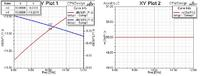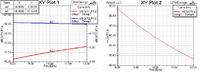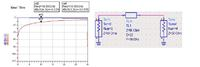# [SOLVED]Waveport renormalize in HFSS

Status
Not open for further replies.

#### qqpost

##### Member level 1Hi,

I have a question about the renormalization. I'm now trying to compare the results of CPW model from ADS to HFSS. When I use the renormalization of the waveport to 50 ohm, the S11 and S21 are much similar to that in ADS (a little difference between them, but I guess they are much close),but the re(Z0(P1)) is always 50 ohm. When I don't use the normalization function, the re(Z0(P1)) is the same as it in ADS according to different structure while S11 and S21 are different.

So, I am just wondering what is the purpose of renormalization? and what is re(Z0(P1)) represent? Just as my guess, the 50 ohm waveport in HFSS seems like the 50 ohm term in ADS. And re(Z0(P1)) is more or less like the characteristic impedance of the transmission line I can get in ADS. Is that right?

Why should we renormalize the waveport? And what does the S11 S21 stand for when non- renormalization for both waveports at the CPW? Is there any meaning for S11 S21 when only renormalize one of the waveports?

Thank you

Last edited:

#### sv1437

##### Full Member level 6Did you simulate the structure without re-normalizing in HFSS ? And see whether there is difference in results (HFSS) ?

If you don't renormalize, HFSS calculates the port impedance based on the width of the line and substrate properties and even the waveport dimensions matter. So one should make sure that width is equal to 50 ohm.

But if you renormalize the port impedance to 50 ohm, no matter whether the feedline is matched to 50 ohm or not, the port impedance is constant at 50 ohm for all the frequencies.

-sv

---------- Post added at 19:26 ---------- Previous post was at 19:23 ----------

And one more thing, if you renormalize (since the port is matched to 50 ohms) you will have good S11. If you don't renormalize and the feed line is not matched to 50 ohm S11 will be poor compared to the one when you renormalized.

•Soni Singh, Rajarshi Bhattacharya and qqpost

Points: 2

Points: 2

### Soni Singh

Points: 2

#### qqpost

##### Member level 1I want use some pictures to show my problem.

In ADS, I can get the S11 of -12db@10GHz and the characteristic impedance of the ideal model is 95ohm. And in HFSS, with renormalization, I can get same S11 but a constant characteristic impedance of 50ohm, which as I set. Without renormalization, the 95ohm is easy to get but at this time what does the S11 stand for?

I know the characteristic impedance is related to the structure of CPW, that is what you mean by get the 50 ohm without renormalization by restricting the width of CPW. Yes?

And one more thing I cannot understand. You said "S11 will be poor compared to the one when you renormalized". I understand partially. However, In ADS simulation, the transmission line impedance is, like in my example, 95 ohm, which shares difference with term impedance of 50ohm. They are not match. But here in HFSS, will S11 be poor? and is there any physical meaning of this poor S11?

And in what situation that should we consider the renormalization function?

Thank you so much.

Here are the pictures.
First one is the result in HFSS with renormalization of WavePort to 50 ohm.Second one is the result in HFSS without renormalization.This is my model in ADS.---------- Post added at 15:40 ---------- Previous post was at 15:25 ----------

And also I found a phenomenon. When changed the condition of renormalization, HFSS will not rerun the simulation but get the result immediately. So that's why I guess there are no complicate calculation between the renormalization. If so, can we get the characteristic impedance of the transmission line by directly calculating from the S parameter? I know that S matrix and Z matrix can change to each other easily. But Z matrix is not the same as Z0. While, how does the HFSS work when deal with the renormalization?

Last edited:

#### cpiresc

##### Newbie level 1Hi,

I have the same problem on my simulation. Are you find the explanation for this problem?

#### qqpost

##### Member level 1Hi cpiresc,

You need to do the renormalization (50 ohm) to get the S-para.

#### ace_operations

##### Junior Member level 3As already mentioned in this thread, if you renormalize your waveport impedance to 50 ohms, you make your driving port impedance independent of waveport size, substrate, and transmission line width. In this case, you can calculate the input impedance looking through one port by considering the 95 ohm transmission line to be terminated by a 50 ohm load. You can then calculate S11 as 20log(|(Zin - 50ohms)/(Zin + 50 ohms)|). This is exactly the case simulated in ADS, and the result of HFSS with renormalization should be same as the ADS model.

When you don't normalize the waveport impedance, the driving port impedance depends on the waveport size, substrate, and transmission line width, and in this case comes out to ~90ohms. Now you can calculate the input impedance looking through one port by considering the 95 ohm transmission line to be terminated by a ~90 ohm load. You can then calculate S11 as 20log(|(Zin - 90ohms)/(Zin + 90 ohms)|). You will see that the S11 value now is more matched. This is because your Zin value is very close to 90 ohms.

In conclusion, S11 physically indicates how much energy is reflected due to mismatch between the input impedance of the port and the driving impedance of the port. In general, it is not true that un-normalized HFSS results will result in poor matching. It depends on the problem at hand.

Hope this helps!

•rijubrata, buaacc_phd, akhiyat.2 and 4 others

Points: 2

Points: 2

### rijubrata

Points: 2

#### flanelloThe renormalization is a post-process step. Thus it is made without recalculating the fields.

#### caoshuxun

##### Newbie level 4As already mentioned in this thread, if you renormalize your waveport impedance to 50 ohms, you make your driving port impedance independent of waveport size, substrate, and transmission line width. In this case, you can calculate the input impedance looking through one port by considering the 95 ohm transmission line to be terminated by a 50 ohm load. You can then calculate S11 as 20log(|(Zin - 50ohms)/(Zin + 50 ohms)|). This is exactly the case simulated in ADS, and the result of HFSS with renormalization should be same as the ADS model.

When you don't normalize the waveport impedance, the driving port impedance depends on the waveport size, substrate, and transmission line width, and in this case comes out to ~90ohms. Now you can calculate the input impedance looking through one port by considering the 95 ohm transmission line to be terminated by a ~90 ohm load. You can then calculate S11 as 20log(|(Zin - 90ohms)/(Zin + 90 ohms)|). You will see that the S11 value now is more matched. This is because your Zin value is very close to 90 ohms.

In conclusion, S11 physically indicates how much energy is reflected due to mismatch between the input impedance of the port and the driving impedance of the port. In general, it is not true that un-normalized HFSS results will result in poor matching. It depends on the problem at hand.

Hope this helps!

Excellent explain, and That really help a lot. Thank you!

•Soni Singh

### Soni Singh

Points: 2

#### ruchak

##### Junior Member level 2hi,
I want to fabricate my design and i m getting very good s11 pattern after renormalization. The results were not satisfactory without renormalization, should i proceed with the fabrication???

#### ruchak

##### Junior Member level 2As already mentioned in this thread, if you renormalize your waveport impedance to 50 ohms, you make your driving port impedance independent of waveport size, substrate, and transmission line width. In this case, you can calculate the input impedance looking through one port by considering the 95 ohm transmission line to be terminated by a 50 ohm load. You can then calculate S11 as 20log(|(Zin - 50ohms)/(Zin + 50 ohms)|). This is exactly the case simulated in ADS, and the result of HFSS with renormalization should be same as the ADS model.

When you don't normalize the waveport impedance, the driving port impedance depends on the waveport size, substrate, and transmission line width, and in this case comes out to ~90ohms. Now you can calculate the input impedance looking through one port by considering the 95 ohm transmission line to be terminated by a ~90 ohm load. You can then calculate S11 as 20log(|(Zin - 90ohms)/(Zin + 90 ohms)|). You will see that the S11 value now is more matched. This is because your Zin value is very close to 90 ohms.

In conclusion, S11 physically indicates how much energy is reflected due to mismatch between the input impedance of the port and the driving impedance of the port. In general, it is not true that un-normalized HFSS results will result in poor matching. It depends on the problem at hand.

Hope this helps!

hi,
I want to fabricate my design and i m getting very good s11 pattern after renormalization. The results were not satisfactory without renormalization, should i proceed with the fabrication???

Status
Not open for further replies.